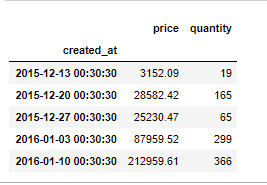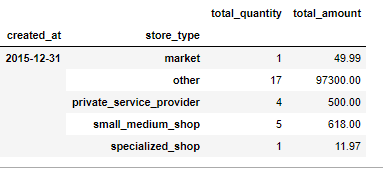Related Articles

# How to group data by time intervals in Python Pandas?

• Last Updated : 26 Dec, 2020

Prerequisites: Pandas

Grouping data by time intervals is very obvious when you come across Time-Series Analysis. A time series is a series of data points indexed (or listed or graphed) in time order. Most commonly, a time series is a sequence taken at successive equally spaced points in time.

Pandas provide two very useful functions that we can use to group our data.

• resample()— This function is primarily used for time series data. It is a Convenience method for frequency conversion and resampling of time series. Object must have a datetime-like index (DatetimeIndex, PeriodIndex, or TimedeltaIndex), or pass datetime-like values to the on or level keyword. Resampling generates a unique sampling distribution on the basis of the actual data.

Syntax : DataFrame.resample(rule, how=None, axis=0, fill_method=None, closed=None, label=None, convention=’start’, kind=None, loffset=None, limit=None, base=0, on=None, level=None)

Parameters :

• rule : the offset string or object representing target conversion
• axis : int, optional, default 0
• closed : {‘right’, ‘left’}
• label : {‘right’, ‘left’}
• convention : For PeriodIndex only, controls whether to use the start or end of rule
• loffset : Adjust the resampled time labels
• base : For frequencies that evenly subdivide 1 day, the “origin” of the aggregated intervals. For example, for ‘5min’ frequency, base could range from 0 through 4. Defaults to 0.
• on : For a DataFrame, column to use instead of index for resampling. Column must be datetime-like.
• level : For a MultiIndex, level (name or number) to use for resampling. Level must be datetime-like.

• Grouper — Grouper allows the user to specify on what basis the user wants to analyze the data.

Syntax: dataframe.groupby(pd.Grouper(key, level, freq, axis, sort, label, convention, base, Ioffset, origin, offset))

Parameters:

• key: selects the target column to be grouped
• level: level of the target index
• freq: groupby a specified frequency if a target column is a datetime-like object
• axis: name or number of axis
• sort: to enable sorting
• label: interval boundary to be used for labeling, valid only when freq parameter is passed.
• convention: If grouper is PeriodIndex and freq parameter is passed
• base: works only when freq is passed
• Ioffset: works only when freq is passed
• origin: timestamp to adjust grouping on the basis of
• offset: offset timedelta added to the origin

### Approach

• Import module
• Resample the data as required
• Grouping the data

Implementation using this approach is given below:

Dataframe in use: timeseries.csv

Program : Aggregating using resampling

## Python3

 `import` `numpy as np``import` `pandas as pd`` ` `# loading dataset``data ``=` `pd.read_csv(``'path of dataset'``)`` ` `# setting the index for the data``data ``=` `data.set_index([``'created_at'``])`` ` `# converting index to datetime index``data.index ``=` `pd.to_datetime(data.index)`` ` `# Changing start time for each hour, by default start time is at 0th minute``data.resample(``'W'``,  loffset``=``'30Min30s'``).price.``sum``().head(``2``)``data.resample(``'W'``, loffset``=``'30Min30s'``).price.``sum``().head(``2``)`` ` `# we can also aggregate it will show quantity added in each week``# as well as the total amount added in each week``data.resample(``'W'``, loffset``=``'30Min30s'``).agg(``    ``{``'price'``: ``'sum'``, ``'quantity'``: ``'sum'``}).head(``5``)`

Output:Program : Grouping the data based on different time intervals

In the first part we are grouping like the way we did in resampling (on the basis of days, months, etc.) then we group the data on the basis of store type over a month Then aggregating as we did in resample It will give the quantity added in each week as well as the total amount added in each week.

## Python3

 `import` `numpy as np`` ` `import` `pandas as pd`` ` `# loading dataset`` ` `data ``=` `pd.read_csv(r``'path of dataset'``)``# setting the index for the data``data ``=` `data.set_index([``'created_at'``])``# converting index to datetime index``data.index ``=` `pd.to_datetime(data.index)`` ` `# Changing start time for each hour, by default start time is at 0th minute``data.resample(``'W'``,  loffset``=``'30Min30s'``).price.``sum``().head(``2``)``data.resample(``'W'``, loffset``=``'30Min30s'``).price.``sum``().head(``2``)`` ` `data.groupby([pd.Grouper(freq``=``'M'``), ``'store_type'``]).agg(total_quantity``=``(``'quantity'``, ``'sum'``),``                                                       ``total_amount``=``(``'price'``, ``'sum'``)).head(``5``)`

Output:Attention geek! Strengthen your foundations with the Python Programming Foundation Course and learn the basics.

To begin with, your interview preparations Enhance your Data Structures concepts with the Python DS Course. And to begin with your Machine Learning Journey, join the Machine Learning – Basic Level Course

My Personal Notes arrow_drop_up